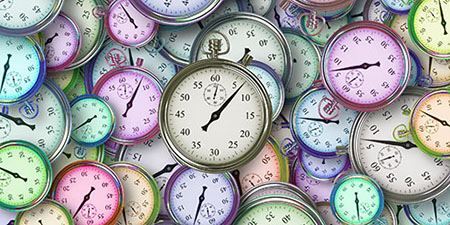# Millisecond (ms) to Nanosecond (ns)

Thema Time units ﹣ Converter Millisecond to Nanosecond

Here you can convert the Time unit Millisecond into the unit Nanosecond and vice versa you can convert Nanosecond into Millisecond. By clicking the "Swap units" icon, you will always obtain the desired conversion in the calculation result, i.e., ms to ns or ns to ms. With the following calculator you can also calculate any other Time unit.

## Info about "Millisecond"

The unit millisecond or thousandth of a second can be traced back to the basic unit second. Here, 1 millisecond corresponds to 0.001 seconds or 1 second corresponds to 1,000 milliseconds. The prefix "milli" in the term millisecond stands for one-thousandth of the base unit second.

This unit belongs to the International System of Units (SI).

For example, while an accuracy of a tenth of a second is sufficient for sprints in athletics to detect the difference between a few centimeters ahead, thousandths of a second in a car race in milliseconds are required to clearly determine the winner.

## Info about "Nanosecond"

The unit “nanosecond” can be traced back to the basic unit second. Therefore, 1 nanosecond corresponds to 0.000000001 seconds or 1 second corresponds to 1,000,000,000 nanoseconds. The prefix "nano" in the term nanosecond stands for one-billionth of the basic unit second.

This unit belongs to the International System of Units (SI).

For example, light travels around 0.3 meters in a nanosecond.

## Basis for conversion Millisecond (ms) to Nanosecond (ns) and vice versa

The abbreviation for the "Time unit Millisecond" is ms. The abbreviation for the "Time unit Nanosecond" is ns.

## Formula for the conversion of Millisecond (ms) to Nanosecond (ns) and vice versa

The calculation from Millisecond to Nanosecond shall be made using the following conversion formula:

Conversion formula Millisecond to Nanosecond

Determine the number of Nanosecond from Millisecond

Millisecond × 1000000

## Formula for the conversion of Nanosecond (ns) to Millisecond (ms)

The calculation from Nanosecond to Millisecond shall be made using the following conversion formula:

Conversion formula Nanosecond to Millisecond

Determine the number of Millisecond from Nanosecond

Nanosecond × 1.0E-6

## Overview table : How many Millisecond are in Nanosecond ?

Millisecond ms ⇒ Nanosecond ns
0.01 ms  are  10 000 ns
0.02 ms  are  20 000 ns
0.03 ms  are  30 000 ns
0.04 ms  are  40 000 ns
0.05 ms  are  50 000 ns
0.06 ms  are  60 000 ns
0.07 ms  are  70 000 ns
0.08 ms  are  80 000 ns
0.09 ms  are  90 000 ns
0.10 ms  are  100 000 ns
0.20 ms  are  200 000 ns
0.30 ms  are  300 000 ns
0.40 ms  are  400 000 ns
0.50 ms  are  500 000 ns
0.60 ms  are  600 000 ns
0.70 ms  are  700 000 ns
0.80 ms  are  800 000 ns
0.90 ms  are  900 000 ns
1 ms  corresponds to  1 000 000 ns
2 ms  are  2 000 000 ns
3 ms  are  3 000 000 ns
4 ms  are  4 000 000 ns
5 ms  are  5 000 000 ns
6 ms  are  6 000 000 ns
7 ms  are  7 000 000 ns
8 ms  are  8 000 000 ns
9 ms  are  9 000 000 ns
10 ms  are  10 000 000 ns
20 ms  are  20 000 000 ns
30 ms  are  30 000 000 ns
40 ms  are  40 000 000 ns
50 ms  are  50 000 000 ns
60 ms  are  60 000 000 ns
70 ms  are  70 000 000 ns
80 ms  are  80 000 000 ns
90 ms  are  90 000 000 ns
100 ms  are  100 000 000 ns
200 ms  are  200 000 000 ns
300 ms  are  300 000 000 ns
400 ms  are  400 000 000 ns
500 ms  are  500 000 000 ns
600 ms  are  600 000 000 ns
700 ms  are  700 000 000 ns
800 ms  are  800 000 000 ns
900 ms  are  900 000 000 ns
1 000 ms  are  1 000 000 000 ns

## Overview table : How many Nanosecond are in Millisecond ?

Nanosecond ns ⇒ Millisecond ms
10 ns  are  0.00001 ms
20 ns  are  0.00002 ms
30 ns  are  0.00003 ms
40 ns  are  0.00004 ms
50 ns  are  0.00005 ms
60 ns  are  0.00006 ms
70 ns  are  0.00007 ms
80 ns  are  0.00008 ms
90 ns  are  0.00009 ms
100 ns  are  0.00010 ms
200 ns  are  0.00020 ms
300 ns  are  0.00030 ms
400 ns  are  0.00040 ms
500 ns  are  0.00050 ms
600 ns  are  0.00060 ms
700 ns  are  0.00070 ms
800 ns  are  0.00080 ms
900 ns  are  0.00090 ms
1 000 ns  are  0.00100 ms

## Source information

As source for the information in the "Time units" category, we have used in particular:

## Last update on May 2, 2022

The pages of the "Time units" category were last editorially reviewed by Stefan Banse on May 2, 2022. They all correspond to the current status.

### Previous changes on April 24, 2021

• April 24, 2021: Publication of the time units converter.
• Editorial revision of all texts in this category